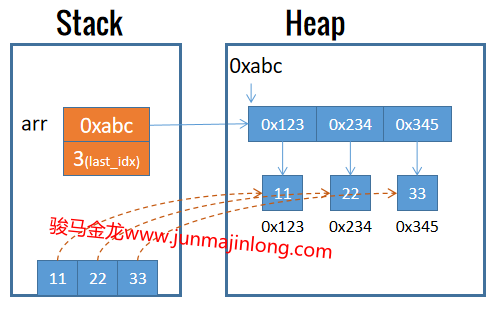## 理解列表

``````my @arr = (11,22,33);
``````

``````my @arr = qw(11,22,33);
``````

``````my \$a = (11,22,33); # \$a=33
``````

### 区分列表和数组

``````my @arr = (11,22,33);  # 数组arr的元素来自于列表
``````